# Functions in C#

## Functions in C# with Examples

In this article, I am going to discuss Functions in C# with Examples. Please read our previous article, where we discussed Goto Statement in C# with Examples. As part of this article, you will understand what methods are and their type and how to create and call functions in C# with Examples.

##### What are the Functions of C# Language?

A function is a group of related instructions that performs a specific task. It can be a small or big task, but the function will perform that task completely. Functions take some input as parameters and return the result as a return value. If we write a function, then we can reuse the function multiple times in the program. That means functions allow us to reuse the code without retyping the code.

##### Why do we need functions?

Let us understand why we need functions with an example. Functions are also called modules or procedures. Instead of writing a single main program, i.e., everything inside the main function, we can break the main function into small manageable size pieces and separate the repeating tasks or smaller tasks as a function.

For Example, if we write one program and put everything inside the main function, then such a type of programming approach is called Monolithic Programming. If your main function contains thousands of lines of code, then it is becoming very difficult to manage. This is actually not a good programming approach.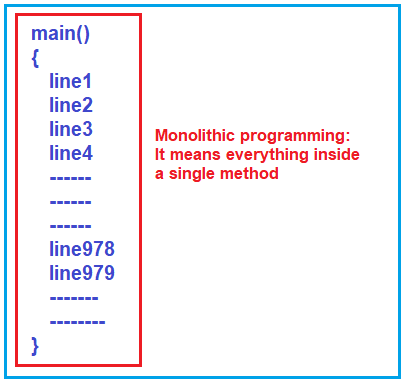##### Problems in Monolithic Programming:
1. First problem: if there is an error in a single line, it’s an error in the entire program or main function.
2. Second problem: 10000 lines of code we cannot finish in one hour or one day; it might take a few days, and we should remember everything throughout that time. Then only we can make changes or write new lines in the program. So, we should memorize the whole program.
3. Third problem: How many people can write this one single main function? Only one person can write. We cannot make it a teamwork and more than one person can’t work on the same main function. So, work cannot be distributed in a team.
4. Fourth problem: when this program becomes very big, it may fit in some computer memories, and it may not fit in some of the memories. It Depends on the size and the hardware contribution of the computer you are running.

So, these are the few problems due to monolithic programming. Monolithic means everything is a single unit.

We prefer to break the program into pieces, manageable and small pieces, and reusable pieces. The benefit of this is we can develop piece-wise so we can concentrate on one piece of code at a time. The second thing is that pieces can be distributed among the team of programmers, and they can develop some pieces, and we can collect them together and make it a single program.

So, suppose we break the program into smaller tasks, i.e., into many smaller functions, and each function performs a specific task. In that case, such type of programming is called “modular programming” or “procedural programming,” and this approach is good for development.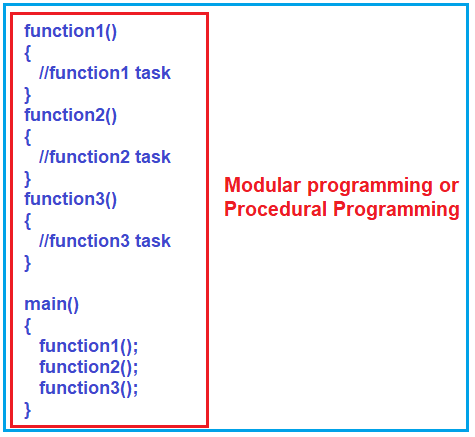As shown in the above image, the first function, i.e., function1(), will perform some specific task, and another function, i.e., function2(), will perform some other task, and similarly, function3() may perform some task. So, in this way, we can break the larger task into smaller simple tasks, and then we can use them all together inside the main function.

Here, in the Modular Programming Approach, you can break the program into smaller tasks, focus on smaller tasks, finish them, and make them perfect. It is easy for one single individual to develop the application, even if you can break this software project into a team of programmers where each programmer will focus on one or many smaller tasks.

This Modular style of programming approach has increased productivity and also reusability. For example, if you want the logic of function2 three times inside the main method, then you need to call function2 three times. That means we are reusing the logic defined in function 2. This is called reusability.

##### Types of Functions in C#:

Basically, there are two types of Functions in C#. They are as follows:

1. Built-in Functions
2. User-Defined Functions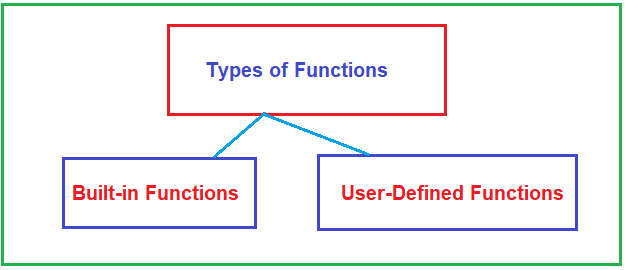Note: The function which is already defined in the framework and available to be used by the developer or programmer is called a built-in function, whereas if the function is defined by the developer or programmer explicitly, then it is called a user-defined function.

##### Advantages of Using Standard Library functions in C# Language:
1. One of the most important reasons you should use library functions or built-in functions is simply because they work. These built-in functions or pre-defined functions have already gone through multiple testing phases and are easy to use.
2. The built-in functions are optimized for performance. So, you will get better performance with built-in functions. Since the functions are “standard library” functions, a dedicated group of developers is constantly working on them to make them better.
3. It saves development time. General functions like printing to a screen, calculating the square root, and many more are already written. You shouldn’t worry about creating them once again. You need to use them and save your time.
##### Example to understand Built-in C# Functions:

In the below example, we are using the built-in WriteLIne function to print the output on the console window and the built-in Sqrt function to get the square root of a given number.

```using System;
namespace FunctionDemo
{
class Program
{
static void Main(string[] args)
{
int number = 25;
double squareRoot = Math.Sqrt(number);
Console.WriteLine(\$"Square Root of {number} is {squareRoot}");
}
}
}
```

Output: Square Root of 25 is 5

##### What are the limitations of Pre-Defined Functions in C# Language?

All the predefined functions in C# are contained limited tasks only, i.e., for what purpose the function is designed for the same purpose it should be used. So, whenever a predefined function does not support our requirements, we need to go for user-defined functions.

##### What are User-Defined Functions in C# Language?

The User-defined functions in C# are the functions that are created by the programmer so that he/she can use them many times. It reduces the complexity of a big program and optimizes the code. C# allows you to define functions according to your need. The function whose body is implemented by the developer or user is called a user-defined function.

As per client or project requirements, the functions we are developing are called user-defined functions. Always user-defined functions are client-specific functions or project-specific functions only. As a programmer, we have full control of user-defined functions. As a programmer, it is possible to alter or modify any user-defined function’s behavior if required because the coding part is available.

##### Advantages of User-Defined Functions in C#:
1. The application code will be easier to understand, maintain, and debug.
2. We can write the code once, and we can reuse the code in many places, i.e., code reusability.
3. Program size reduced. As the duplicate code is put inside a single function, the size of the application code is going to be reduced.
##### How to Create a User-Defined Function in C#?

Let us see how to write a function in C#. First of all, the function should have a name that is mandatory. Then it should have a parameter list (the parameters it is taking) which is optional; then the function should have a return type which is mandatory. A function can have an access specifier, which is optional, and a modifier which is also optional. For a better understanding, please have a look at the below image.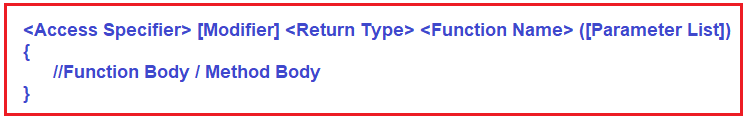Here,

1. Function Name: It is mandatory, and it defines the name of the method or function. The method signature consists of the method name and parameter list. The Methods are identified by their name. The rules for giving function names are the same as the rules for giving variable names. Same rules you should follow for giving function names also.
2. Parameter List: It is Optional and defines the list of parameters. A function that can take 0 or more parameters may not take any input.
3. Return Type: It is Mandatory and defines the method’s return type value. A function may or may not return a value, but it can return at most one value. It cannot return multiple values but can take multiple values as parameters. If the function is not returning any value, then the return type should be void.
4. Access Specifier: It is Optional and defines the method’s scope. That means it defines the accessibility of the method, such as private, protected, public, etc.
5. Modifier: It is optional and defines the method’s access type. For example, static, virtual, partial, sealed, etc. If you declare the method with a static modifier, then you can access the method directly without creating an instance. If you declare the method with the sealed modifier, then this method is not going to be overridden under a child class. And if you declare the method with the partial modifier, then you can split the method definition into two parts.
6. Function Body: The function’s body defines the code or list of statements you need to execute the function call. It is enclosed within curly braces.

Note: Access Specifiers and Modifiers are not the same. Method and Function are both the same, so we can use the term Method and Function interchangeability.

##### Example to Create User-Defined Function in C#: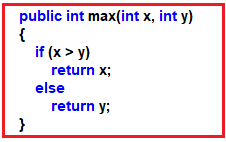In the above example,
public is the access specifier
int is the return type
max is the Method Name
(int x, int y) is the Parameters list
And this method does not have any modifiers.

##### What is Function Signature in C#?

In C# programming language, a Method Signature is consisting of two things, i.e., the Method Name and the Parameter List. The return type is not considered to be a part of the method signature. Later we will discuss why the return type is not considered part of the method signature.

###### Example to Understand Function Signature in C#: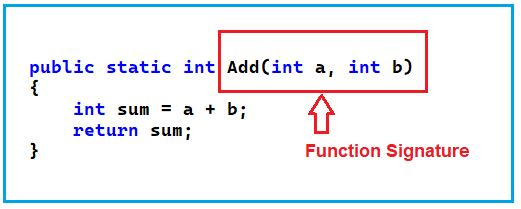##### What is Return Statement in C#?

The return statement terminates the execution of a function immediately and returns the control to the calling function. Execution resumes in the calling function at the point immediately following the call. A return statement can also return a value to the calling function. A return statement causes your function to exit and return a value to its caller. In general, the function takes inputs and returns some value. The return statement is used when a function is ready to return a value to its caller.

##### How to Call a Method in C#?

When a method is invoked (called), a request is made to perform some action, such as setting a value, printing statements, performing some complex calculation, doing some database operation, returning some data, etc. The code we need to invoke a method contains the name of the method to be executed and any data the receiving method requires. The required data for a method is specified in the method’s parameter list.

When we invoke a method, the control gets transferred to the called method. Then the called method returns the control to the caller method (from where we call the method) in the following three conditions.

1. When the return statement is executed.
2. When it reaches the method ending closing curly brace.
3. When it throws an exception that is not handled in the called method.
##### Example to Understand Functions in C# Language:

Let us see how to create and call a method in C#. In the below example, we have implemented the logic to add two numbers and then print the result on the console window, and we have written the logic inside the main method only.

```using System;
namespace FunctionDemo
{
class Program
{
static void Main(string[] args)
{
int x, y;
x = 10;
y = 15;
int sum = x + y;
Console.WriteLine(\$"Sum is {sum}");
}
}
}
```

As you can see in the above code, first, we declare two variables, x and y, and then we initialize these two variables with values 10 and 15, respectively. Then we add these two variables and store the result in another variable, i.e., sum, and finally print the sum value in the console, which is what you can see in the output. Let us see how to write the same Program using Function. For a better understanding, please have a look at the below image.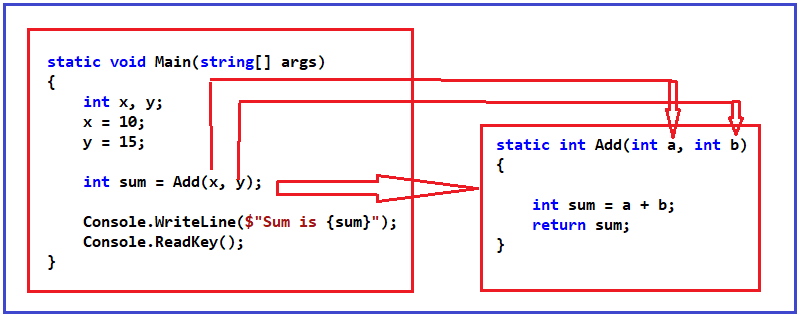As you can see in the above image, we created a function called Add, which takes two input parameters, a and b, of type integer. This Add function adds the two integer numbers it received as input parameters, stores the result in variable sum, and returns the result.

Now see the main function. From the main function, we are calling the Add function. While calling the Add function, we pass two parameters, i.e., x and y (actually, we pass the values stored in x and y). These parameters’ values will go into the a and b variables. The Add function then adds these two values and returns the result to the calling function (the function from where the Add method is called), i.e., the Main method. The main function then stores the result from the Add method into the variable sum and prints the result on the output window.

##### Complete Example Code is Given Below:
```using System;
namespace FunctionDemo
{
class Program
{
static void Main(string[] args)
{
int x, y;
x = 10;
y = 15;
Console.WriteLine(\$"Sum is {sum}");
}

static int Add(int a, int b)
{
int sum = a + b;
return sum;
}
}
}
```
##### Different Parts of a Function in C#:

To understand the different parts of a function, please look at the image below.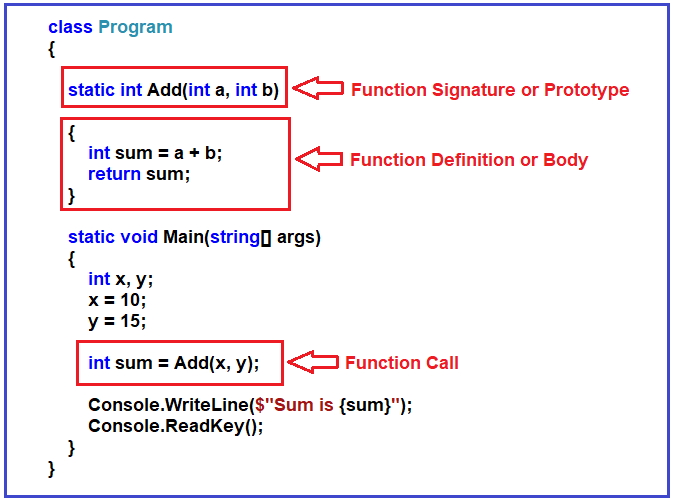##### What are the Parameters of a function?

For a better understanding of the function parameters, please look at the image below.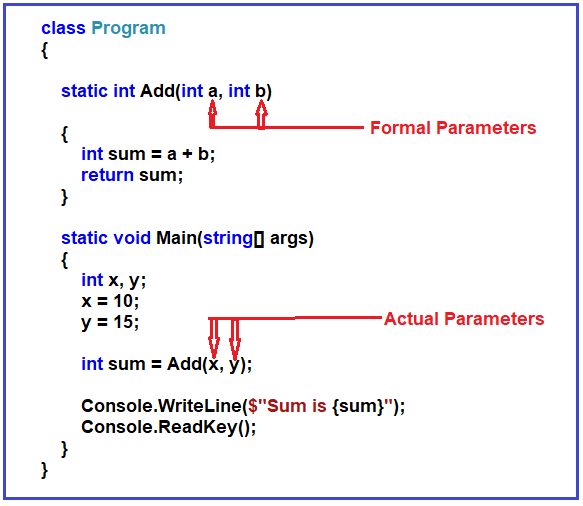As you can see in the above image, we are passing two values, x, and y, to the Add function, which takes two parameters (a and b). The parameters (x and y) that we are passing to the Add function are called Actual Parameters. The parameters (a and b) which are taken by the Add method are called Formal Parameters. When we call the Add method, the values of actual parameters are copied to the formal parameters. So, the x value, i.e., 10, is copied to a, and the y value, i.e., 15, is copied to b.

##### How does it work inside the Main memory?

When the program starts, i.e., when the main method starts its execution, three variables (x, y, and sum) are declared inside the stack, that is, inside the activation area of the main function. Then the x and y are assigned with values 10 and 15, respectively. And then, the main method calls the Add method. Once the Add method is called, its own activation area is created inside the stack, and it will have its own variables, i.e., variables a, b, and sum are created inside this activation area. Then the value of x i.e. 10, and the value of y i.e. 15, passed to Add function, are copied to the variables a and b, respectively. Then the Add method adds the two numbers and the result is 25, which is stored in the variable sum, and that result, i.e., 25, is returned from the Add method. That result coming from the Add method is stored in the variable sum, and that will be printed in the console window. For a better understanding, please have a look at the following image.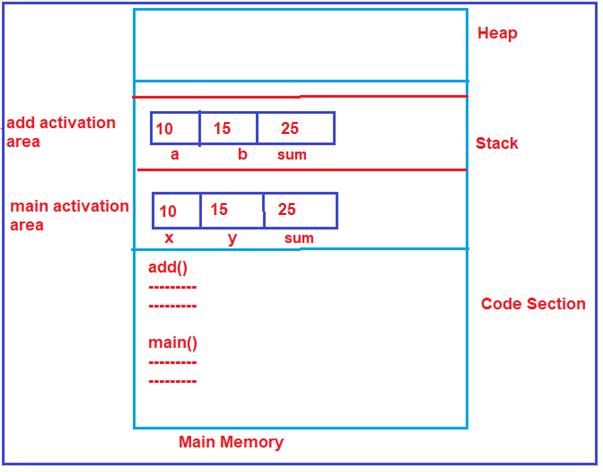So, this is what happens inside the main memory when we are writing functions. One more point you need to remember is that one function cannot access the variables of other functions. I hope you understand the basics of Functions in the C# language.

1.2.